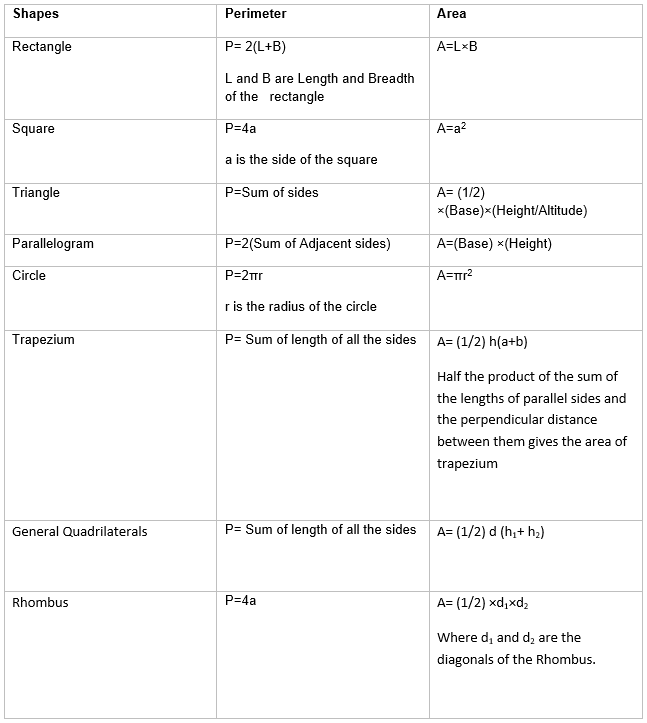# Mensuration class 8 notes

In this page we will explain the topics for the chapter 11 of Mensuration Class 8 Maths.We have given quality Mensuration class 8 notes along with video to explain various things so that students can benefits from it and learn maths in a fun and easy manner, Hope you like them and do not forget to like , social share and comment at the end of the page.
Table of Content

## Mensuration

It is branch of mathematics which is concerned about the measurement of length, area and Volume of plane and Solid figure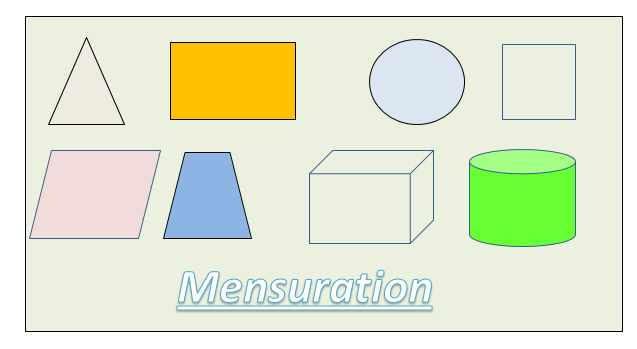## Perimeter & Area of Common & Known Shapes

Perimeter
• The perimeter of plane figure is defined as the length of the boundary
• It units is same as that of length i.e. m ,cm,km
 1 Meter 10 Decimeter 100 centimeter 1 Decimeter 10 centimeter 100 millimeter 1 Km 10 Hectometer 100 Decameter 1 Decameter 10 meter 1000 centimeter

Area
• The area of the plane figure is the surface enclosed by its boundary
• It unit is square of length unit. i.e. m2 ,  km2
 1 square Meter 100 square Decimeter 10000 square centimeter 1 square Decimeter 100 square  centimeter 10000 square  millimeter 1 Hectare 100 square  Decameter 10000 square meter 1 square myraimeter 100 square kilometer 108 square meter

### Shapes where Area and Perimeter are known

 Shapes Perimeter Area Rectangle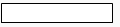P= 2(L+B) L and B are Length and Breadth of the   rectangle A=L×B Square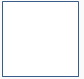P=4a a is the side of the square A=a2 Triangle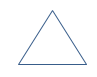P=Sum of sides A=(1/2)×(Base)×(Height/Altitude) Parallelogram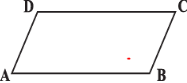P=2(Sum of Adjacent sides) A=(Base) ×( Height) Circle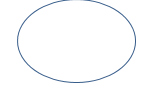P=2πr r is the radius of the circle A=πr2

Watch this tutorial for example around Perimeter

## Area of Trapezium

Trapezium is a quadrilateral with a pair of parallel sides.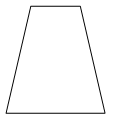Isosceles trapezium
Trapezium when non-parallel sides of it are of equal length
Area of the Trapezium can be found by dividing the trapezium into two parts of three parts depending
On the shapes of the trapezium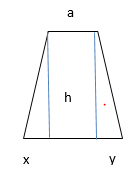(So two triangles and One rectangle)
Here h is the distance between the parallel sides
a   and b are the parallel sides
A= (1/2)hx + (1/2)hy + ah
=(1/2)h( x+y+ 2a)
Now b=x+y+a
So
=(1/2)h( a+b)
Similarly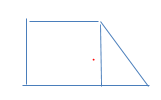(One triangle and One rectangle)
It can be proved easily here also
A=(1/2)h( a+b)
So to find the area of a trapezium we need to know the length of the parallel sides and the
perpendicular distance between these two parallel sides. Half the product of the sum of
the lengths of parallel sides and the perpendicular distance between them gives the area of
trapezium
Example
A trapezoid's two bases are 10 m and 6m, and it is 4m high. What is its Area?
Solution:
A=(1/2)h( a+b)
So
A= (1/2)4(10+6)
=32 m2

We can always divide a general quadrilateral into two triangles by drawing the diagonal. This split the quadrilateral into two triangles and Sum of areas of the triangles is equal to the area of the quadrilaterals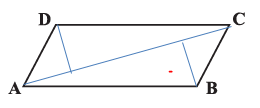Here is the above figure, Diagonal AC divides the quadrilateral ABCD into two triangles.  We have drawn perpendicular to this diagonal from opposite vertices. They are of height h1 and h2 respectively
So Area of Quadrilateral ABCD will be given as
=Area of the Triangle ACD + Area of the Triangle ABC
=(1/2)×AC×h1 + (1/2)×AC×h2
=(1/2)AC(h1+ h2)
=(1/2)d(h1+ h2)
d is the length of the diagonal

We already know the formula for the area of special quadrilaterals (Rectangle, square and parallelogram). Those can also be derived from the above general quadrilaterals formula
We can similarly derive the formula for the Rhombus
Area of Rhombus is given by
A= (1/2)×d1×d2
Where d1 and d2 are the diagonals of the Rhombus.
Examples
1) The area of a rhombus is 240 cm2  and one of the diagonals is 10 cm. Find the other diagonal.
Solution:
Area of Rhombus is given by
A= (1/2)×d1×d2
240=(1/2)10×d
d=45 cm

## Area of Polygons

We will encounter figures which are having more than 4 sides.  We can derive the area of the polygons by using the same approach i.e. dividing into triangles or quadrilaterals

## Solid Shapes

Solid shapes are three dimensional figures.  We can identify common two dimensional figures on there faces
Example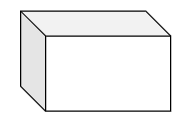It is a cube which has square shapes on the all the faces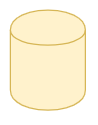It is cylinder and has circular figure at two bases

## Volume,Surface area in case of Solid Figures

 Surface Area Surface area of a solid is the sum of the areas of its faces Lateral Surface Are The faces excluding the top and bottom) make the lateral surface area of the solid Volume Amount of space occupied by a three dimensional object (Solid figure) is called its volume. we use square units to find the area of a two dimensional region. In case of volume we will use cubic units to find the volume of a solid, as cube is the most convenient solid shape (just as square is the most convenient shape to measure area of a region) Volume is sometimes refer as capacity also
Volume Units
 1 cm3 1mL 1000 mm3 1 Litre 1000ml 1000 cm3 1 m3 106 cm3 1000 L 1 dm3 1000 cm3 1 L

## How to find the surface Area and Volume of the solid Figures

Surface Area is the area of the faces. So we need to find the area of the each of the faces and sum them to find the total surface area
In-case of Cuboid, all the faces are rectangles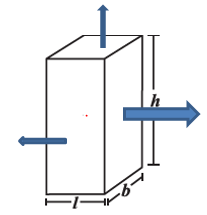Surface Area
=2×(Area of the rectangle having length and Breath  as h and b  )+2×(Area of the rectangle having length and Breath  as l and b  ) +2×(Area of the rectangle having length and Breath  as l and h  )
=2( LB + BH + LH ).
Volume is the space occupied by the solid figure. We can measure it by counting cubes in the space.
if the base and top of the solid are congruent and parallel to each other and its edges are perpendicular to the base then volume can be deduced as
Volume of solid=area of base × height

## Surface Area and Volume of Cube and Cubiod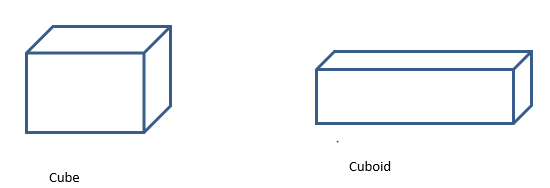Type Measurement Surface Area of Cuboid of Length L, Breadth B and Height H 2( LB + BH + LH ). Lateral surface area of the cuboids 2( L + B ) H Diagonal of the cuboids $\sqrt {L^2 + B^2 + H^2}$ Volume of a cuboids LBH Length of all 12 edges of the cuboids 4 (L+B+H). Surface Area of Cube of side L 6L2 Lateral surface area of the cube 4L2 Diagonal of the cube $L \sqrt {3}$ Volume of a cube L3

## Surface Area and Volume of Right circular cylinder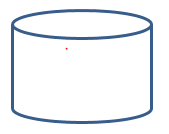Radius The radius (r) of the circular base is called the radius of the cylinder Height The length of the axis of the cylinder is called the height (h) of the cylinder Lateral Surface The curved surface joining the two base of a right circular cylinder is called Lateral Surface.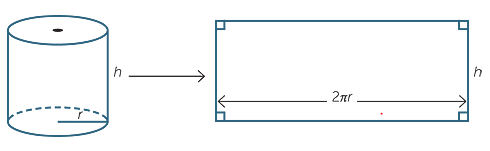Type Measurement Curved or lateral Surface Area of cylinder 2πrh Total surface area of cylinder 2πr (h+r) Volume of Cylinder π r2h

Example:
Find the height of a cylinder whose radius is 14 cm and the total surface area is 1936 cm2
Assume π= 22/7
Solution
Let height of the cylinder = h, radius = r = 14cm
Total surface area = 2πr (h + r)
2× (22/7)× 14 × (h + 14) = 1936
h = 8 cm

Example
Given a cuboid tank, in which situation will you find surface area and in
which situation volume.
(a) To find how much water it can hold.
(b) Number of cement bags required to plaster it.
(c) To find the number of smaller tanks that can be filled with water from it.

Solution
(a) Volume as we are talking about capacity
(b) Surface area
(c) Again Volume

## Summary

Here is Mensuration Class 8 Notes Summary